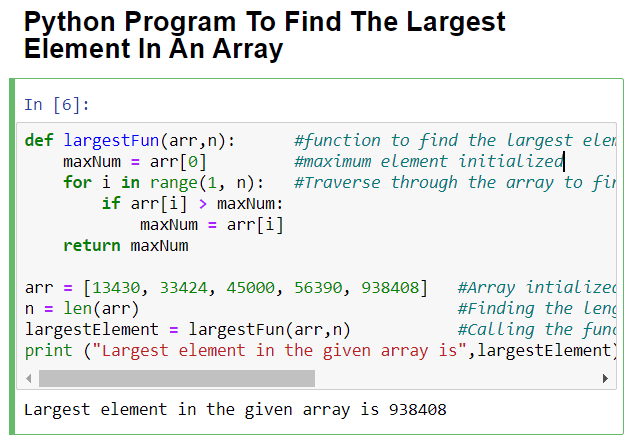# Python 3 Program To Find Largest Element In An Array or List

3755In this tutorial, you will learn a simple Python 3 program to find the largest element in an array or list using functions. Before proceeding further, you must be aware of the basics of Python programming language, such as how to use variables, create functions, etc. If you have just begun your Python journey, then do check out these recommended books.

## Python 3 program to find the largest element in an array or list

To find the largest element in an array, we will simply create a function called largestFun() with two arguments. The first argument will take the array, and the second argument will take the length of the array (for traversing the array).

Inside the function, we will initialize a variable named maxNum to store the maximum value or the largest element of the passed array. Next, we will use the Python For loop to traverse the array and comparing every element of the array with the value stored in the variable.

Once the array is traversed completely, maxNum will store the value of the largest element in the array. We will return the maxNum value from the function. Now, we will initialize an array with some integer values and find its length using the len() function.

In the end, we will call the largestFun() and store the value returned in the variable called largestElement and print its value to display the maximum number in an array or list.

Please refer to the code below, and if you have any doubts, then share them in the comments so that I can assist you.

Source Code:

```def largestFun(arr,n):      #function to find the largest element in an array with two arguements - array and size of array.
maxNum = arr         #maximum element initialized
for i in range(1, n):   #Traverse through the array to find the largest element
if arr[i] > maxNum:
maxNum = arr[i]
return maxNum

arr = [13430, 33424, 45000, 56390, 938408]   #Array intialized
n = len(arr)                                 #Finding the length of array
largestElement = largestFun(arr,n)           #Calling the function to find the largest element in the array.
print ("Largest element in the given array is",largestElement) #Printing output.```

### Program Output